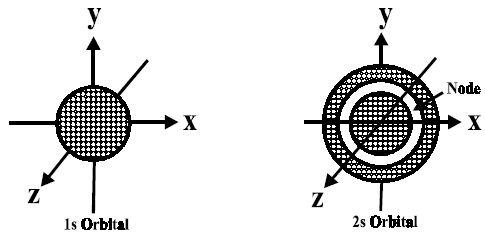# Describe on S-Orbitals

For s-orbitals, when l = 0, the value of m is 0; i.e., there is only one possible orientation. This means that the probability of finding an electron is the same in all directions at a given distance from the nucleus. It should, therefore, be spherical in shape. Hence all s- orbitals are non- directional and spherically symmetrical about the nucleus.

The size of an s-orbital depends upon the value of the principal quantum number n. Greater the value of ‘n’ larger is the size of the orbital.Fig: Shapes of 1s and 2s-orbitals

An important feature of the 2s-orbital is that there is a spherical shell within this orbital where the probability of finding the electron is zero (nearly). This is called a node or nodal surface. In 2s orbital, there is one spherical node. The number of nodal surfaces or nodes in s-orbital of any energy level is equal to (n-1), where n is the principal quantum number.